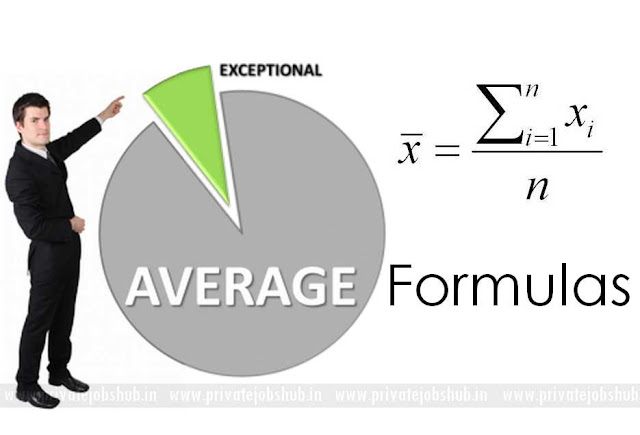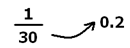## Average Formula

Candidates who are going to take participate in government exams; they should have knowledge about, how many questions will be asked in the exam from the particular chapter. Here we will discuss about Average Formula and its important shortcut tricks and methods, because average topic is one of the important topics in quant section. Dear candidates, you all know that calculation speed sets the base for Quantitative aptitude section in various exams. If the candidates know sufficient Tricks in Quantitative aptitude Section then they will definitely achieve better in the section.

So, on this page we are providing you important shortcut tricks, formula of average and method to solve the question. We have tried to make it easy for all the students through easy methods and tricks on average and these shortcuts will save your time as well and will be helpful in upcoming government exams. These questions will be useful to score good marks. Please have a look.

What is Average?

The end result obtained by adding numerous quantities jointly and then dividing this total by number of quantities, it is called Average. The main expression of average is equivalent allocation of a value amongst all which may distribute persons or things. Here is average based some fact and formula and some average shortcut Maths tricks examples. The problem is given in Quantitative Aptitude which is a very essential paper in bank exam. Formula:

Average: = (Sum of observations / Number of observations).

Some Important Examples

Examples 1: what will be the average of 13, 14, 15, 16, and 17?

Solution: Average is the middle term when the number of terms is odd, but before that let’s checks whether it is in A.P or not, since the common difference is same so the series is in A.P. So the middle term is 15 which is our average of the series.

Example 2: What will be the average of 13, 14, 15, 16, 17, and 18?

Solution: We have discussed that when the number of terms are even then the average will be the average of two middle terms.

Now the two middle terms are 15 and 16, but before that the average we must check that the series should be A.P. Since the common difference is same for each of the term we can say that the series is in A.P. and the average is (16+15)/2 = 15.5

 Take a Test  Now

Example 3: The average of five numbers is 29. If one number is excluded the average becomes 27. What is the excluded number?

Answer: Let the excluded number is
= (29 x 5) – (27 x 4)
= 145 – 108
= 37

Example 4: Find the average of first 20 natural numbers?

Sum of first n natural numbers = n (n + 1) /2
So, we can find easily average of first 20 natural numbers 20 x 21 / 2 = 210
So, then Required average is = 210 / 20 = 10.5.

Example 5 Find the average of first 20 multiplies of 5.

Required average = 5 (1 + 2 + 3 +……………….. + 20) /20
= (5 x 20 x 21 / 20 x 2) = 2100 / 40 = 52.5
So the Required average is 52.5.

Some Important Questions:

Question: The average age of 30 boys of a class is equal to 14 yrs. When the age of the class teacher is included the average becomes 15 yrs. Find the age of the class teacher.

Solution: Total ages of 30 boys = 14 × 30 = 420 yrs.
Total ages when class teacher is included = 15 × 31 = 465 yrs.
Age of class teacher = 465 - 420 = 45 yrs.

Direct Formula:

Age of new entrant = New average + No. of old members × increase in average

= 15 + 30 (15 – 14) = 45 yrs.

Question: The average weight of 4 men is increased by 3 kg when one of them who weigh 120 kg is replaced by another man. What is the weight of the new man?

Solution:  Quicker approach: If the average is increased by 3 kg, then the sum of weighs increases by 3 × 4 = 12 kg. And this increase in weight is due to the extra weight included due to the inclusion of new person.

Weight of new person = 120 + 12 = 132 kg.

Direct formula: Weight of new person = weight of removed person + No. of persons × increase in average = 120 + 12 × 3 = 132 kg.

Question: The average of 11 results is 50. If the average of first six results is 49 and that of last six is 52, find the sixth result.

Solution: The total of 11 results = 11 × 50 = 550 and the total of first 6 results = 6 × 49 = 294, the total of last 6 results = 6 × 52 = 312
The 6th result is common to both; Therefore, Sixth result = 294 + 312 – 550 = 56

Question: The average of a batsman in 16 innings is 36. In the next innings, he is scoring 70 runs. What will be his new average?

a) 44
b) 38
c) 40
d) 48

Solution: Conventionally solving:

New average = (old sum+ new score)/ (total number of innings) = ((16 ×36) +70)/ ((16+1)) = 38

Shortcut technique:

Step 1) Take the difference between the new score and the old average = 70-36= 34
Step 2) This 34 extra runs is spread over 17 innings = 34/17=2
Step 3) Hence, the average increases by 2 =>36+2 = 38 is the new average

Question: The average marks of 19 children in a particular school are 50. When a new student with marks 75 joins the class, what will be the new average of the class?

Solution:
Step 1) Take the difference between the old average and the new marks = 75-50=25
Step 2) This score of 25 is distributed over 20 students => 25/20 = 1.25
Step 3) Hence, the average increases by 1.25=> 50+1.25 = 51.25.

Question: The average age of Mr. Mark’s 3 children is 8 years. A new baby is born. Find the average age of all his children?

Solution:
The new age will be 0 years. The difference between the old average and the new age = 0-8= -8
This age of 8 years is spread over 4 children => (-8/4= -2) Hence, the average reduces to 8-2= 6 years.
Now here is a technique which will help to compute the new value when the average is given.

Question: The average age of 29 students is 18. If the age of the teacher is also included the average age of the class becomes 18.2.Find the age of the teacher?

Solution:
a) 28
b) 32
c) 22
d) 24

Conventionally solving,
Let the average age of the teacher =x
(29 × 18 + x × 1)/30
Solving for x, we get x = 24.
Using the shortcut, based on the same method we used previously
Step 1: calculate the change in average = 18.2-18 = 0.2.
This change in 0.2 is reflected over a sample size of 30.The new age is increased by 30 * 0.2 = 6 years above the average i.e. 18+6= 24, which is the age of the teacher.

Question: The average of four numbers is 80. The first number is 1 / 3 of sum of three numbers. What would be the first number?

Solution: P + Q + R + S = 320 - (I)
P = Q + R + S / 3 - (II)
From (I) and (II)
4P = 240
P = 80

Question: In a college, 16 girls have the average age is 18 years and 14 boys have the average age 17 years. What would be the average age of entire college?

Solution: 16 girls has the average age is 18 years (16 x 18) = 288
4 boys has the average age 17 years (14 x 17) = 238
Average age is = 288 + 238 / 30 = 526 / 30 = 17.54 years

Question: The average of five numbers is 29, if one number is exclude the average becomes 27. What is the exclude number?

Solution: Let the exclude number is
= (29 x 5) – (27 x 4)
= 145 – 108
= 37.
The exclude number 37.

Question: The average of five positive integers is 55.8 If average of first two integers is 49 and the average of fourth and fifth integers is 69.5 Than find the thired integer.

Solution: Shortcut
55.8 x 5 = 279
49 x 2 = 98
69.5 x 2 = 139
Third number = (279 – 98 – 139) = 42

Question: In a School 8 student average weight is increased by 3.5 kg, when new student comes in place of one of them than weight become 55 kg, what would be the weight of the new student ?

Solution:
Step 1: At first we find the total weight increased so, (8X 3.5) kg = 28 kg.
Step 2: Now the weight of new Student is = (55 + 28) kg = 83 kg.
So, the weight of the new student is 83 kg.

Question: The Average weight 3 girls A, B, and C is 55 kg, While the average weight of three boys B, D, and E is 57 kg. What is the average weight of A, B, C, D, E?

Solution:
Step 1: At First we find 3 girls total weight (A + B + C) = (55 X 3) = 165 kg. And Total 3 boys weight of (B + D + E) = (57 x 3) = 171.
Step 2: Adding both weight of (A + 2 B + C + D + E) = (165 + 171) = 336 kg.

Note: So, to find the average weight of A B C D and E, we duty to know B’s weight, which is not given. So the data is inadequate.

 Check Some Other Important Maths Preparation Links

To know more shortcut tricks stay connected with us and subscribe this web page. Candidates can also get exam pattern, syllabus and latest update by visiting to the site www.privatejobshub.com.